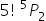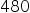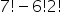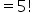Maths-
General
Easy

Question

# The number of ways can 7 boys be seated at a round table so that 2 particular boys are separated is :

##6!2!Hint:

## The correct answer is:### Step by step solution :We want to find the numbers of ways in which 7 boys can be seated on a round table when 2 particular boys are seperated.Let us assume that two boys put together a single unit.Now, we will arrange 6 entities in 6 places on a circular table.When n objects are arranged in n places in a circular table, then there are (n−1)! ways.That is, if there are 6 boys and 6 places, then there are (6−1)!ways to arrange.Now, the two boys that were fixed in a single place can also rearrange themselves.The number of ways in which two boys can arrange among themselves is 2!Next, to find the number of ways 7 boys can be seated at a round table so that 2 particular boys are next to each other is multiply 5! with 2!That is, total ways possible are 5!2!.Total ways of arranging 2 boys sitting together = 5! 2! = 240.Total ways of arranging 7 boys at a round table = (7–1)! = 6! = 720Total number of ways in which they are separated  = Total ways of arranging 7 boys at a round table - Total ways of arranging 2 boys sitting togetherThus, Total number of ways in which they are separated  = 720 - 240 = 480

When n distinct objects are arranged in n places, then the total number of ways possible are n!. But, when n objects are to arranged in n places on a circular table, there are (n1)!ways.#### With Turito Foundation.#### Get an Expert Advice From Turito.